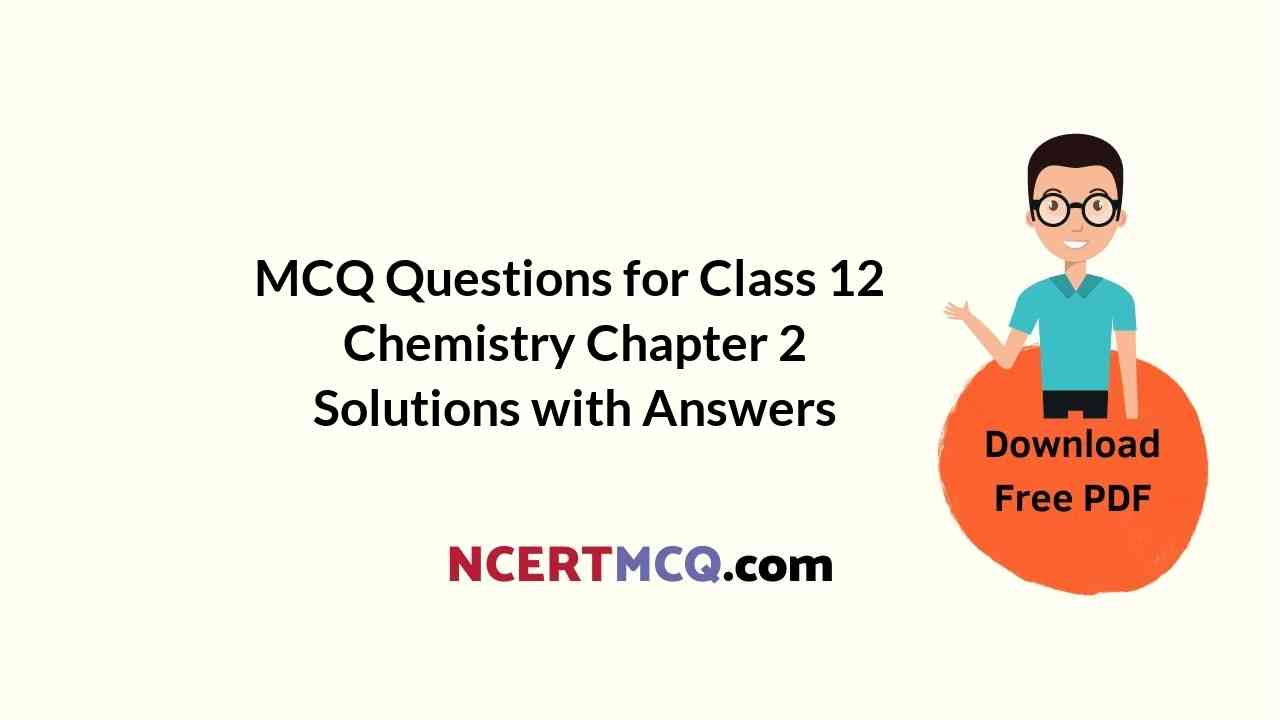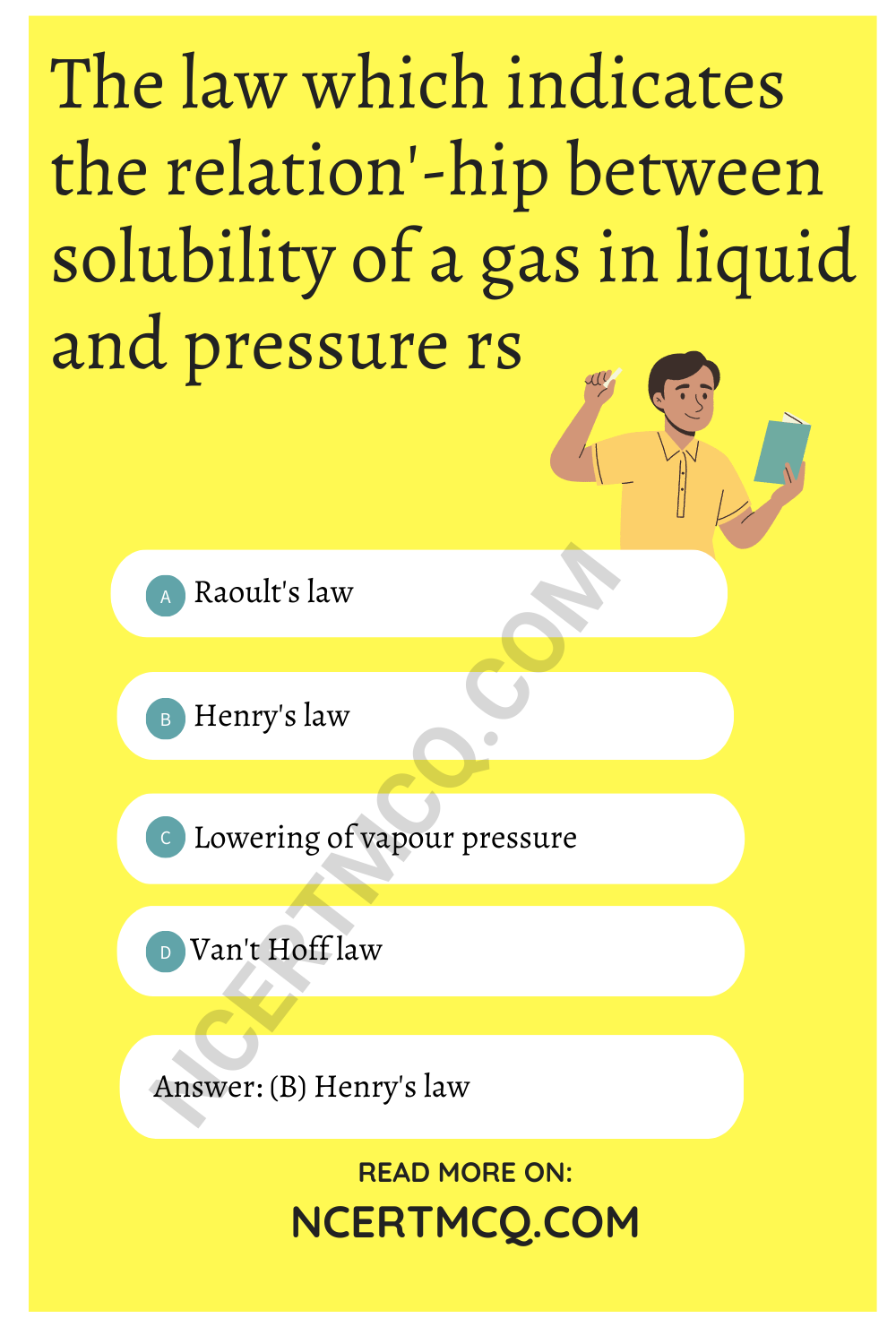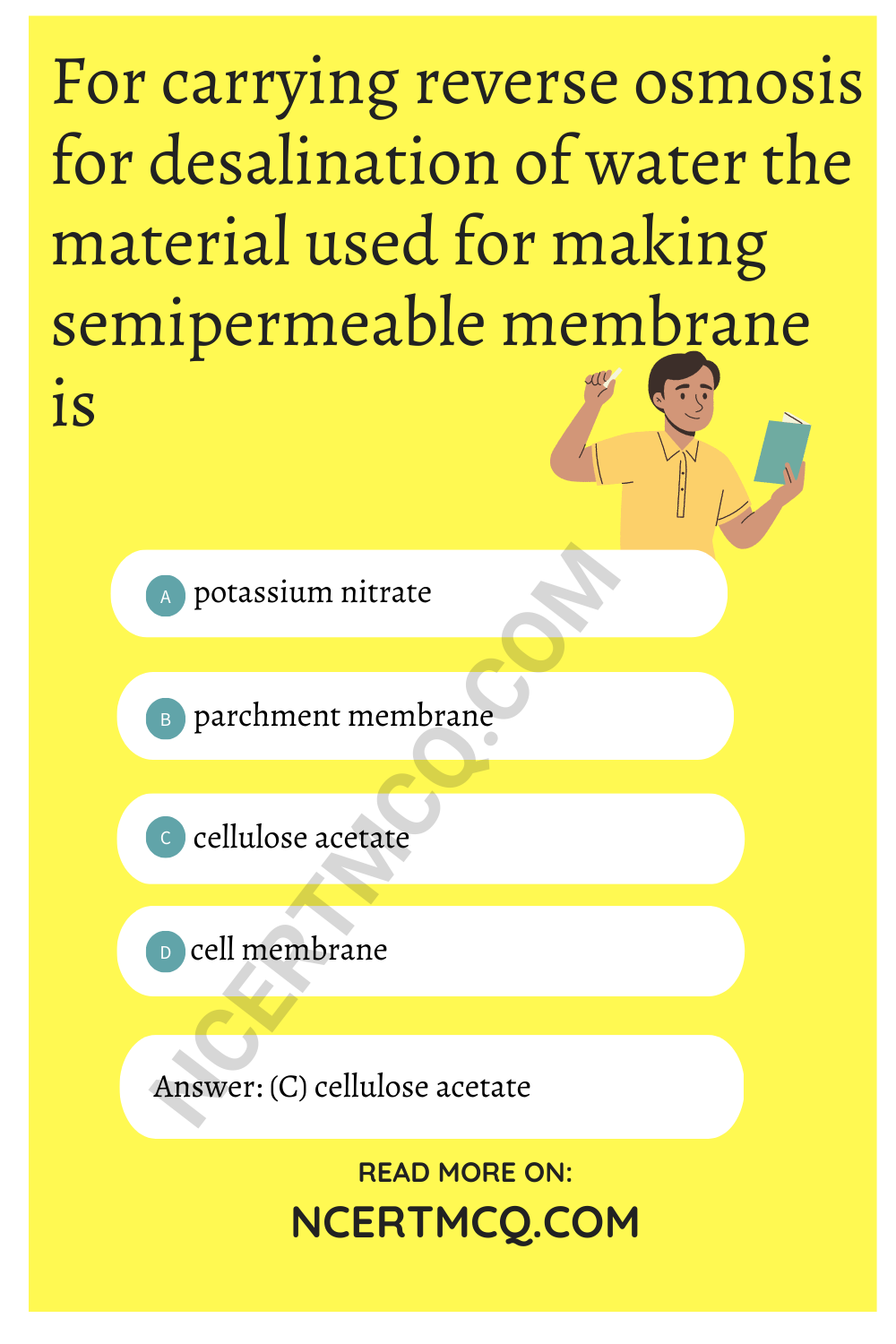Check the below NCERT MCQ Questions for Class 12 Chemistry Chapter 2 Solutions with Answers Pdf free download. MCQ Questions for Class 12 Chemistry with Answers were prepared based on the latest exam pattern. We have provided Solutions Class 12 Chemistry MCQs Questions with Answers to help students understand the concept very well.

## Solutions Class 12 MCQs Questions with Answers

Solutions Class 12 MCQ Question 1.
If 2 gm of NaOH is present is 200 ml of its solution, its molarity will be
(a) 0.25
(b) 0.5
(c) 5
(d) 10

MCQ On Solutions Class 12 Question 2.
The atmospheric pollution is generally measured in the units of
(a) mass percentage
(b) volume percentage
(c) volume fraction
(d) ppm

Solution MCQ Class 12 Question 3.
A 5% solution of cane-sugar (molecular weight = 342) is isotonic with 1% solution of substance A. The molecular weight of X is
(a) 342
(b) 171.2
(c) 68.4
(d) 136.8

Class 12 Chemistry Chapter 2 MCQ Question 4.
234.2 gm of sugar syrup contains 34.2 gm of sugar. What is the molal concentration of the solution.
(a) 0.1
(b) 0.5
(c) 5.5
(d) 55

MCQ Of Chapter 2 Chemistry Class 12 Question 5.
H2S is a toxic gas used in qualitative analysis. If solubility of H2S in water at STP is 0.195 m. what is the value of KH?
(a) 0.0263 bar
(b) 69.16 bar
(c) 192 bar
(d) 282 bar

Class 12 Chemistry Chapter 2 MCQs Question 6.
Atomic mass is equal to
(a) number of electrons of an atom
(b) sum of the numbers of electrons and protons of an atom
(c) sum of the numbers of neutrons and protons of an atom
(d) none of these

Answer: (b) sum of the numbers of electrons and protons of an atom

MCQ Questions For Class 12 Chemistry Chapter 2 Question 7.
Henry’s law constant for molality of methane is benzene at 298 K is 4.27 × 105 mm Hg. The mole fraction of methane is nenzene at 298 K under 760 mm Hg is
(a) 1.78 × 10-3
(b) 17.43
(c) 0.114
(d) 2.814

Class 12 Solutions MCQ Question 8.
How much oxygen is dissolved in 100 rnL water at 298 K if partial pressure of oxygen is 0.5 atm and K = 1.4 × 10-3 mol/L/atm?
(a) 22.4 mg
(b) 22.4 g
(c) 2.24 g
(d) 2.24 mg

MCQ Solutions Class 12 Question 9.
The law which indicates the relation’-hip between solubility of a gas in liquid and pressure rs
(a) Raoult’s law
(b) Henry’s law
(c) Lowering of vapour pressure
(d) Van’t Hoff lawMCQ Of Solution Chapter Question 10.
Among the following substances the lowest vapour pressure is exerted by
(a) water
(b) alcohol
(c) ether
(d) mercury

MCQ On Solution Chapter Class 12 Question 11.
Vapour pressure of a pure liquid X is 2 atm at 300 K. It is lowered to 1 atm on dissolving 1 gof Y in 20 g of liquid X. If molar mass of X is 200, what is the molar mass of Y?
(a) 20
(b) 50
(c) 100
(d) 200

Chapter 2 Chemistry Class 12 MCQ Question 12.
Partial pressure of a solution component is directly proportional to its mole fraction. This is known as
(a) Henry’s law
(b) Raoult’s law
(c) Distribution law
(d) Ostawald’s dilution law

Solution Class 12 MCQ Question 13.
3 moles of P and 2 moles of Q are mixed, what will be their total vapour pressure in the solution if their partial vapour pressures are 80 and 60 torr respectively?
(a) 80 torr
(b) 140 torr
(c) 72 torr
(d) 70 torr

Solutions Chemistry Class 12 MCQ Question 14.
Which of the following solutions shows positive deviation from Raoult’s law?
(a) Acetone + Aniline
(b) Acetone + Ethanol
(c) Water + Nitric acid
(d) Chloroform + Benzene

MCQ Questions On Solutions In Chemistry Class 12 Question 15.
The system that forms maximum boilingazetrope is
(a) Acetone-chloroform
(b) ethanol-acetone
(c) n-hexane-n-heptane
(d) carbon disulphide-acetone

Question 16.
A plant cell shrinks when it is kept in a
(a) hypotonic solution
(b) hypertonic solution
(c) isotonic solution
(d) pure water

Question 17.
The relative lowering in vapour pressure is proportional to the ratio of number of
(a) solute molecules to solvent molecules
(b) solvent molecules to solute molecules
(c) solute molecules to the total number of molecules in solution
(d) solvent molecules to the total number of molecules in solution

Answer: (c) solute molecules to the total number of molecules in solution

Question 18.
A solution containing 10.2 g glycerine per litre is isotonic with a 2% solution of glucose. What is the molecular mass of glycerine?
(a) 91.8 g
(b) 1198 g
(c) 83.9 g
(d) 890.3 g

Question 19.
What weight of glycerol should be added to 600 g of water in order to lower its freezing point by 10°C ?
(a) 496 g
(b) 297 g
(c) 310 g
(d) 426 g

Question 20.
The osmotic pressure of a solution can be increased by
(a) increasing the volume
(b) increasing the number of solute molecules
(c) decreasing the temperature
(d) removing semipermeable membrane

Answer: (b) increasing the number of solute molecules

Question 21.
Sprinkling of salt helps in clearing the snow covered roads in hills. The phenomenon involved in the process is
(a) lowering in vapour pressure of snow
(b) depression in freezing point of snow
(c) melting of ice due to increase in temperature by putting salt
(d) increase in freezing point of snow

Answer: (b) depression in freezing point of snow

Question 22.
For carrying reverse osmosis for desalination of water the material used for making semipermeable membrane is
(a) potassium nitrate
(b) parchment membrane
(c) cellulose acetate
(d) cell membraneQuestion 23.
What will be the degree of dissociation of 0.1 M Mg(NO3)2 solution if van’t Hoff factor is 2.74?
(a) 75%
(b) 87%
(c) 100%
(d) 92%

Question 24.
Which of the following units is useful in relating concentration of solution with its vapour pressure?
(a) Mole fraction
(b) Parts per million
(c) Mass percentage
(d) Molality

Question 25.
At equilibrium the rate of dissociation of a solid solute in a volatile liquid solvent is
(a) less than the rate of crystallisation
(b) greater than the rate of crystallisation
(c) equal to the rate of crystallisation
(d) zero